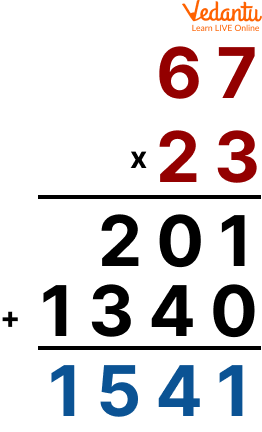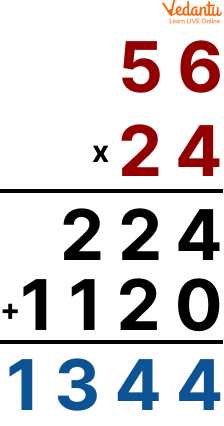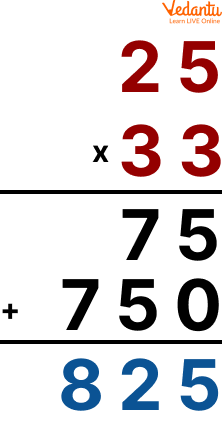Courses
Courses for Kids
Free study material
Free LIVE classes
More

# Multi-Step Word ProblemsLIVE
Join Vedantu’s FREE Mastercalss

## Overview of Multi-Step Word Problems

As we know, in Mathematics, multiplication is one of the four basic operations; the others are addition, subtraction and division. Multiplication is known as the repeated addition of the same number. Likewise, double-digit multiplication is simply single-digit multiplication which is done twice. It is done by arranging the numbers so that the provided numbers are placed one below the other.

A 2-digit number can be multiplied with a single digit, with another 2-digit number or a 3-digit number and so on. Therefore, in this chapter, we will learn about 2-digit by 2-digit multiplication, multi-step word problems, and word problems on addition and subtraction.

## What is 2-Digit Multiplication?

2-digit multiplication is the method or process of multiplying 2-digit numbers arranged in two place values, i.e., ones and tens. The process of multiplying numbers is the same as multiplying single digits. However, in 2-digit multiplication, we multiply each digit by one by the multiplier. It means the multiplier is first multiplied with the one digit of the multiplicand and then multiplied with the tens digit of the multiplicand.An example of 2-digit multiplication

## 2-Digit by 2-Digit Multiplication Multi-Step Word Problems

2-digit by 2-digit multiplication multi-step word problems are mathematical problems that involve multiple steps and require the use of 2-digit by 2-digit multiplication. These problems can be challenging because they require students to not only perform mathematical calculations but also to understand and interpret the problem and to use that information to determine the correct solution. In this, we need to convert the statements into a mathematical form, and then we can multiply them.

Here, we will have the question in the statement form. Let us understand it with the help of an example:

Example: 24 folders each has 56 sheets of paper inside them. How many sheets of paper are there altogether?

Ans: The multiplication starts with the ones place first and then moves on to the tens place. The numbers are placed one below the other.

We can add 56 sheets 24 times

Or

Since multiplication is repeated addition, we can multiply 56 and 24 to get the answer.Multiplication of 56 and 24

## Solved Examples of Multi-Step Word Problems

Q1. A baker has 57 loaves of bread. He wants to sell each loaf for \$35. How much money will the baker make from selling all the loaves of bread?

Ans: To solve this problem, students would need to perform the 2-digit by 2-digit multiplication of 57 x 35, which is 1995. They would then need to understand that the problem is asking for the total amount of money the baker will make from selling all the loaves of bread and that the answer is \$1995.Multiplication

Thus the product of 57 and 35 is 1995.

Q2. At a party, Sam ate 25 chocolates, each containing 33 calories. At the party, how many calories does Sam consume?

Ans: Write the given numbers in column form. First, multiply the one digit of the multiplier with the multiplicand and then multiply the tens digit with the multiplicand. Add the partial products to obtain the required result.Multiplication

Thus Sam ate a total of 825 calories at the party.

## Practice Problems

Q 1. A teacher has 41 students in her class. Each student needs 32 pages of notebook paper for the school year. How many pages of notebook paper will the teacher need to provide for the class?

Ans: 1312

Q 2. A construction company has 35 workers on its payroll. Each worker needs 16 sets of safety equipment for the construction season. How many sets of safety equipment will the company need to provide for its workers?

Ans: 560

## Word Problems on Addition and Subtraction

Word problems on addition and subtraction are very important to build upon the basic skills among the students. In math, word problems mean that the information is presented through storytelling, and students have to understand it and then transform it into math equations. The worksheets help students to practice and make their basic skills stronger.

Q1. 49 fourth graders, 35 fifth graders and 76 sixth graders showed up for soccer practice. How many students were there in total?

Ans: 160 Students

Q2. A large tank truck can hold up to 11,256 gallons of oil. If the truck already contains 9,365 gallons of oil, how many more gallons will be required to fill the tanker? ​

Ans: 1891 Gallons

## Addition Subtraction Multiplication Division Worksheets

An addition, subtraction, multiplication, and division worksheet is given below:

Q 1. A store has 45 apples, and they received 12 more apples. How many apples do they have now?

Ans: 57

Q 2. A boy had 89 candies, and he ate 12 candies. How many candies he has now?

Ans:

Q 3. A store has 6 shelves of books, and each shelf has 9 books. How many books are there in total?

Ans: 54

Q 4. A shop has 96 chocolates and wants to divide them into boxes of 8 chocolates each. How many boxes will be needed?

Ans: 12

## Summary

So, in this chapter, we have learned and discussed what 2-digit multiplication is and how 2-digit is multiplied by 2-digit in a stepwise process to understand and learn it better. We have solved a few multi-step word problems related to 2-digit by 2-digit multiplication.

We also solved addition subtraction multiplication division worksheets PDF to enhance the skills of the students and make the concept very clear. Apart from that, under this chapter, we will practice worksheets based on the word problems on addition and subtraction so that they can be understood better.

Last updated date: 26th Sep 2023
Total views: 69.3k
Views today: 1.69k

## FAQs on Multi-Step Word Problems

1. What is a Partial Outcome?

While multiplying when we separate one of the numbers into tens, hundreds and so on and then the final product separately with each part. Lastly, add them together to find the multiplication product. For example, multiplying 22 x 5, then

22 x 5 = 20 x 5  + 2 x 5

= 100 + 10 (Partial Outcome)

= 110

2. How to do 2-digit multiplication by 1-digit?

2-digit multiplication by 1-digit is a process of multiplying a 2-digit number by a 1-digit number. For e.g. 23 x 4

Multiply the ones digit of the 2-digit number (in this case 3) by the 1-digit number (in this case 4) and write down the result (3 x 4 = 12). Multiply the tens digit of the 2-digit number (in this case 2) by the 1-digit number (in this case 4) and write down the result (2 x 4 = 8). Add the two products obtained in steps 2 and 3. In this case 12 + 8 = 20

Write down the final result, 92.

3. What are the use of word problems in daily life?

Word problems are an important tool for teaching mathematical concepts and problem-solving skills. They are used daily to help individuals think critically and make decisions based on mathematical information. For example, a word problem may ask how much of each ingredient is needed to make a recipe for 6 people instead of 4.